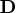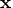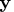Previous Page | Next Page

 Language Reference

Comparison Operators:   <   >   =   <=   >=   ^=

compare matrix elements

matrix1<matrix2
matrix1<=matrix2
matrix1>matrix2
matrix1>=matrix2
matrix1=matrix2
matrix1^=matrix2

The comparison operators compare two matrices element by element and produce a new matrix that contains only zeros and ones. If an element comparison is true, the corresponding element of the new matrix is 1. If the comparison is not true, the corresponding element is 0. You cannot use the English equivalents GT and LT for the greater than and less than signs as you can in Base SAS software. Scalar or row or column vector values can be used instead of matrices in any of the preceding forms. If either operand is a scalar, the operation is performed for that scalar with each element of the matrix. If either operand is a row or column vector, then the operation is performed using that vector on each of the rows or columns of the matrix.

For example, let

a={1 7 3,
6 2 4};
b={0 8 2,
4 1 3};

Evaluation of the following expression yields the matrix, as shown:

c=a>b;

C             2 rows      3 cols    (numeric)

1         0         1
1         1         1

In addition to comparing conformable matrices, you can apply the comparison operators to a matrix and a scalar. If either argument is a scalar, the comparison is between each element of the matrix and the scalar.

For example, the following expression produces the matrix, as shown:

d=(a>=2);

D             2 rows      3 cols    (numeric)

0         1         1
1         1         1

If the element lengths of two character operands are different, the shorter elements are padded on the right with blanks for the comparison.

If a numeric missing value occurs in an operand, IML treats it as lower than any valid number for the comparison.

When you are making conditional comparisons, all values of the result must be nonzero for the condition to be evaluated as true.

Consider the following statement:

if x>=y then goto loop1;

The GOTO statement is executed only if every element ofis greater than or equal to the corresponding element in. See also the descriptions of the ALL and ANY functions.Previous Page | Next Page | Top of Page